# What is the Law of Conservation of Linear Momentum

The law of conservation of linear momentum states that the total momentum of a system of particles remains constant, so long as no external forces act on the system. Equivalently, one could also say that the total momentum of a closed system of particles remains constant. Here, the term closed system implies that there are no external forces acting on the system.

This holds true even if there are internal forces between particles. If a particle$A$ exerts a force$F_{BA}$ on a particle$B$, then the particle$B$ would exert a force of$F_{AB}=-F_{BA}$ on$A$. The these two forces are Newton’s third law pairs, and so they would act for the same duration of time$\Delta t$. The change in momentum for particle$B$ is$\Delta \vec{p_B}=F_{BA}\Delta t$. For particle$A$, the change in momentum is$\Delta \vec{p_A}=F_{AB}\Delta t=-F_{BA}\Delta t=-\Delta \vec{p_B}$. The total change in momentum  within the system is indeed$\Delta \vec{p_B}+\left(-\Delta \vec{p_B}\right)=0$.

## Law of Conservation of Linear Momentum when Two Bodies Collide in 1 Dimension

Suppose an object of mass$m_1$ is traveling with a velocity$u_1$ and another object with mass$m_2$ is traveling with a velocity$u_2$. If these two collide, and then the body with mass$m_1$ started traveling at a velocity$v_1$ and the body with mass$m_2$ started traveling at a velocity$v_2$, according to law of conservation of momentum,Law of Conservation of Linear Momentum – 1D two-body collision$p_{before}=p_{after}\Rightarrow m_1u_1+m_2u_2=m_1v_1+m_2v_2$.

Note that for these cases, the correct direction of velocities need to be put into equations. For instance, if we select the direction to the right to be positive for the above example,$v_1$ would have a negative value.

## Law of Conservation of Linear Momentum when a Body Explodes in 1 Dimension

In explosions, a body breaks into several particles. Examples include firing a bullet from a gun or a radioactive nucleus spontaneously emitting an alpha particle. Suppose a body having a mass$M$, sitting at rest, breaks into two particles having masses$m_1$ which travels at a speed$v_1$, and$m_2$ which travels at a speed$v_2$.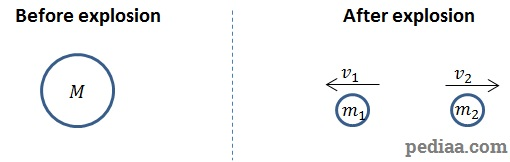Law of Conservation of Linear Momentum – 1D Explosion

According to the law of conservation of momentum,$p_{before}=p_{after}$. Since the initial particle was at rest, its momentum is 0. This means that the momenta of the two smaller particles must also add up to 0. In this case,$m_1v_1+m_2v_2=0$

Again, this would only work if velocities are added along with the correct directions.

## Law of Conservation of Linear Momentum in 2 and 3 Dimensions

The law of conservation of linear momentum applies to 2 and 3 dimensions as well. In these cases, we break up momentum into their components along the$x-$,$y-$ and$z-$ axes. Then, the components of momentum along each direction are conserved. For example, suppose two colliding bodies have momenta$\vec{p_{1i}}=\left(p_{1xi},p_{1yi},p_{1zi}\right)$ and$\vec{p_{2i}}=\left(p_{2xi},p_{2yi},p_{2zi}\right)$ before collision, and momenta$\vec{p_{1f}}=\left(p_{1xf},p_{1yf},p_{1zf}\right)$ and$\vec{p_{2f}}=\left(p_{2xf},p_{2yf},p_{2zf}\right)$ after collision, then,$p_{1xi}+p_{2xi}=p_{1xf}+p_{2xf}$$p_{1yi}+p_{2yi}=p_{1yf}+p_{2yf}$$p_{1zi}+p_{2zi}=p_{1zf}+p_{2zf}$

If the momenta before collision and momenta after collision are all shown in the same vector diagram, they would form a closed shape. For example, if 3 bodies moving in a plane have momenta$p_{1i}$$p_{2i}$ and$p_{3i}$ before collision and momenta$p_{1f}$$p_{2f}$ and$p_{3f}$ after collision, once these vectors are added diagrammatically, they will form a closed shape: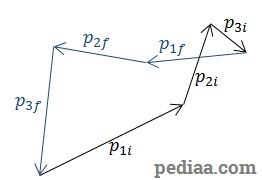Law of Conservation of Linear Momentum – Momentum vectors before and after collision, added together, form a closed shape

## Elastic Collision – Conservation of Momentum

In a closed system, the total energy is always conserved. However, during collisions, some of the energy may be lost as thermal energy. As a result, the total kinetic energy of the colliding bodies may reduce during a collision.

In elastic collisions, the total kinetic energy of the colliding bodies before the collision is equal to the total kinetic energy of the bodies after the collision.

In reality, most collisions that we experience in everyday life are never perfectly elastic, but collisions of smooth, hard spherical objects are nearly elastic. For these collisions, then you have,$m_1u_1+m_2u_2=m_1v_1+m_2v_2$ as well as$\frac{1}{2}m_1{u_1}^2+\frac{1}{2}m_2{u_2}^2=\frac{1}{2}m_1{v_1}^2+\frac{1}{2}m_2{v_2}^2$

Now, we will derive a relationship between the initial and final velocities for two bodies undergoing an elastic collision: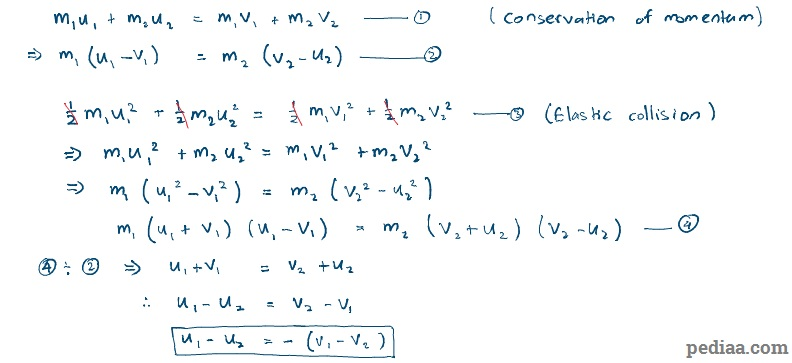Law of Conservation of Linear Momentum – Elastic Collision Velocity Derivation

i.e. the relative velocity between the two objects after an elastic collision has the same magnitude but the opposite direction to the relative velocity between the two objects before the collision.

Let’s now suppose the masses between the two colliding bodies is equal, i.e.$m_1=m_2$. Then our equations become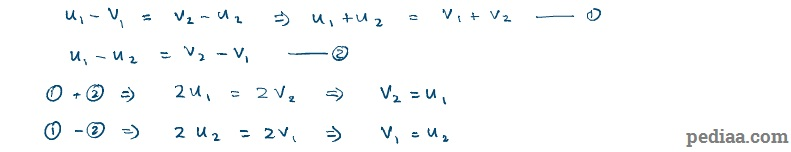Law of Conservation of Linear Momentum – Velocities of Two Bodies After an Elastic Collision

The velocities are exchanged between the bodies. Each body leaves the collision with velocity of the other body before collision.

## Inelastic Collision – Conservation of Momentum

In inelastic collisions, the total kinetic energy of colliding bodies before the collision is less than their total kinetic energy after the collision.

In completely inelastic collisions, the colliding bodies stick together after the collision.

That is, for two colliding bodies during a completely inelastic collision,$m_1u_1+m_2u_2=(m_1+m_2)v$

where$v$ is the velocity of the bodies after collision.

## Newton’s Cradle – Conservation of Momentum

A Newton’s Cradle is the object shown below. It consists of a number of spherical metal balls of equal mass in contact with each other. When any number of balls is raised from one side and let go, they come down and collide with the other balls. After the collision, the same number of balls rises up from the other side. These balls also leave with a velocity equal to that of the incident balls just before the collision.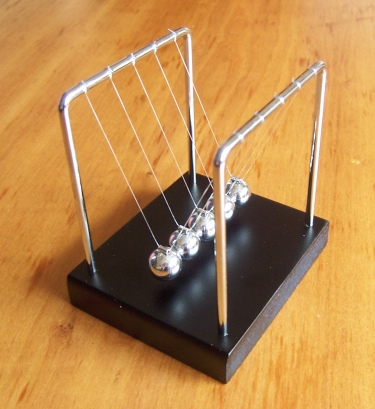What is the Law of Conservation of Linear Momentum – Newton’s Cradle

We can predict these observations mathematically, if we assume the collisions to be elastic. Suppose each ball has a mass$m$. If$p$ is the number of balls initially raised up by a person and$q$ is the number of balls that gets raised as a result of the collision, and if$u$ is the speed of incident balls just before collision and$v$ is the speed of the balls that get raised up after collision,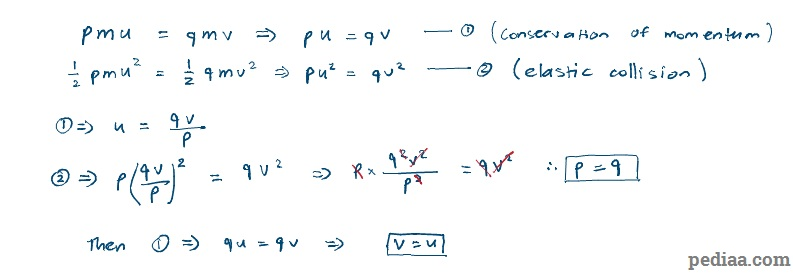What is the Law of Conservation of Linear Momentum – Newton’s Cradle Derivation

i.e. if we raised$n$ balls initially, the same number of balls would get raised after collision.

As the balls are raised, their kinetic energy is converted to potential energy. Considering the conservation of energy, then, the height that the balls rise up to will be same as the height that the balls were raised to by the person.

###### “A Newton’s Cradle” by AntHolnes (Own work) [CC BY-SA 3.0], via Wikimedia Commons